• ## matlab实现带通滤波

万次阅读 2020-07-22 15:19:25
matlab给出的代码如下：[n,Wn] = buttord(Wp,Ws,Rp,Rs) 首先要先理解一个带通滤波器的幅频特性图中，分为通带、阻带、过渡带三大部分。 通带截止频率和阻带截止频率对这三部分进行划分，二者之间的部分即为过渡带...
以带通滤波器——巴特沃斯滤波器为例
matlab给出的代码如下：[n,Wn] = buttord(Wp,Ws,Rp,Rs)
首先要先理解一个带通滤波器的幅频特性图中，分为通带、阻带、过渡带三大部分。
通带截止频率和阻带截止频率对这三部分进行划分，二者之间的部分即为过渡带。
一.如何设计带通滤波器?
1.Wp,Ws分别是通带频率和阻带截止频率
这两个频率是指同一侧的通带和阻带频率，比如设计一个40Hz~100Hz的带通滤波器
Wp1=40Hz,Wp2=100Hz,代表Wp1~Wp2之间是通带
Ws1=20Hz,Ws2=120Hz,代表0~20Hz和120Hz~正无穷为阻带
过渡带宽度=阻带边缘频率-通带边缘频率（高频的一侧）=Ws2-Wp2=20Hz
2.Rp,Rs是描述通带、阻带幅值的两个重要参量
Rp是描述通带波纹（起伏程度）的一个参量，通带纹波是指在滤波器的频响中通带的最大幅值和最小幅值之间的差值，正常的纹波一般小于1db。
通带波纹当然越小越好，这样通带内频率的幅度都基本稳定在单倍幅度上，因此Rp是允许的通带波纹的最大值。
Rs是描述阻带衰减的一个参量
阻带衰减越大越好，衰减越大代表对不想要的信号频率成分的滤除效果越好，因此Rs是允许的需要达到的阻带衰减的最小值。
3.n,Wn是设计得到滤波器的阶数和截止频率
通道滤波器的设计代码例程如下：
Wp = [40 100]/500;
Ws = [20 120]/500;
Rp = 3;
Rs = 40;
[n,Wn] = buttord(Wp,Ws,Rp,Rs)
上述在Wp,Ws的赋值过程中进行了归一化，因此run之后得到的Wn的值也是归一化过的
n = 12
Wn = 0.0799    0.2002
二.如何绘制带通滤波器的幅频响应?
可以先调用butter()函数返回巴特沃斯滤波器传递函数的零极点系数和增益。
[z,p,k] = butter(n,Wn);
sos = zp2sos(z,p,k);

freqz(sos,512,1000)
title(sprintf('n = %d Butterworth Lowpass Filter',n))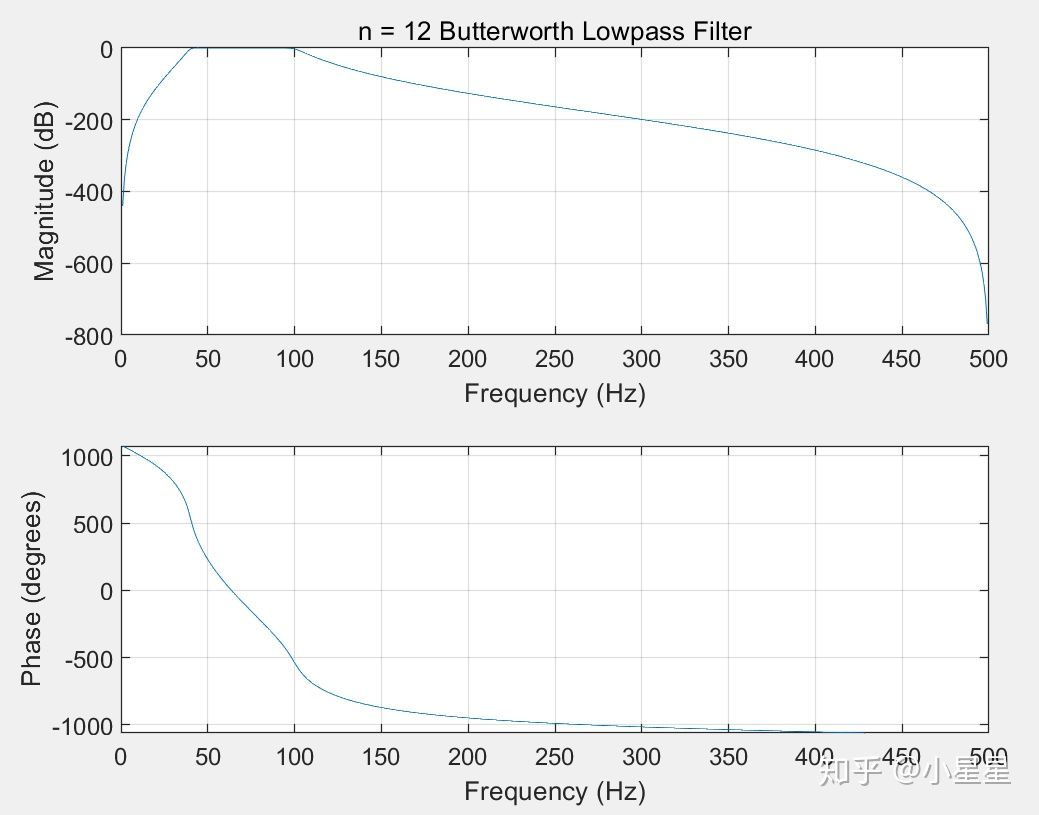三.如何使用带通滤波器对一组数据进行滤波
y = filter(b,a,x) 
利用分子和分母系数 b 和 a 定义的有理传递函数对输入数据 x 进行滤波。
https://zhidao.baidu.com/question/423138525.html
四.如何合理确定滤波器的参数？
我们已知所需要的通带截止频率，阻带截止频率，可以通过一个便捷的方式由matlab直接确定滤波器的参数。输入fdatool命令,具体方法可参见以下博客。
https://blog.csdn.net/qq_42839007/article/details/104184920
https://wenku.baidu.com/view/6bb31c23dcccda38376baf1ffc4ffe473368fd82.html
滤波的阶数
滤波器的阶数，就是滤波几次的意思，一阶，就是对谐波过滤一次，滤波器的阶数越高，滤波效果越好，但是，成本也会成倍的增加，因此，选择合适的阶数是非常重要的。

展开全文信号处理 数字通信 iir滤波器
• ## matlab理想带通滤波

万次阅读 2017-10-20 20:03:19
理想带通使用如下函数，dim是维度，如果input是一维，则dim=1 % FILTERED = ideal_bandpassing(INPUT,DIM,WL,WH,SAMPLINGRATE) % % Apply ideal band pass filter on INPUT along dimension DIM. % % WL: lower ...

理想带通使用如下函数，dim是维度，如果input是一维，则dim=1

% FILTERED = ideal_bandpassing(INPUT,DIM,WL,WH,SAMPLINGRATE)
%
% Apply ideal band pass filter on INPUT along dimension DIM.
%
% WL: lower cutoff frequency of ideal band pass filter
% WH: higher cutoff frequency of ideal band pass filter
% SAMPLINGRATE: sampling rate of INPUT
%
% Copyright (c) 2011-2012 Massachusetts Institute of Technology,
% Quanta Research Cambridge, Inc.
%
% Authors: Hao-yu Wu, Michael Rubinstein, Eugene Shih,
% Date: June 2012
%
function filtered = ideal_bandpassing(input, dim, wl, wh, samplingRate)

if (dim > size(size(input),2))
error('Exceed maximum dimension');
end

input_shifted = shiftdim(input,dim-1);
Dimensions = size(input_shifted);

n = Dimensions(1);
dn = size(Dimensions,2);

Freq = 1:n;
Freq = (Freq-1)/n*samplingRate;
mask = Freq > wl & Freq < wh;

Dimensions(1) = 1;

F = fft(input_shifted,[],1);

filtered = real(ifft(F,[],1));

filtered = shiftdim(filtered,dn-(dim-1));

end


展开全文• %带通滤波 %使用注意事项：通带或阻带的截止频率与采样率的选取范围是不能超过采样率的一半 %即，f1,f3,fs1,fsh,的值小于 Fs/2 %x:需要带通滤波的序列 % f 1：通带左边界 % f 3：通带右边界 % fs1：衰减截止左边界 %...
• ## 带通滤波matlab

千次阅读 2019-01-26 20:02:00
巴特沃斯： 1、带阻滤波器设计 带阻滤波器指标： 阻带上边界频率：5Kz； 阻带下边界频率：7Kz；...巴特沃斯模拟带通滤波器阶数：N=5 转载于:https://www.cnblogs.com/hjj-fighting/p/10324768.html

巴特沃斯：1、带阻滤波器设计带阻滤波器指标：阻带上边界频率：5Kz；阻带下边界频率：7Kz；通带上边界频率：2Kz；通带下边界频率：9Kz；通带最大衰减：1dB；阻带最小衰减：20dB；设计程序如下：wp=2*pi*[2000,9000];ws=2*pi*[5000,7000];Rp=1;As=20;[Nb,wc]=buttord(wp,ws,Rp,As,'s');[BSB,ASB]=butter(Nb,wc,'stop','s');[hk,w]=freqs(BSB,ASB);subplot(2,2,1)plot(w,20*log(abs(hk)),'b')xlabel('频率/Hz')ylabel('幅度/dB')title('幅频特性')subplot(2,2,2)plot(angle(hk))xlabel('频率/Hz')ylabel('相位')title('相频特性')程序运行结果：巴特沃斯模拟带阻滤波器阶数：Nb=42、带通滤波器的设计带通滤波器指标：通带上边界频率：4Kz；通带下边界频率：7Kz；阻带上边界频率：2Kz；阻带下边界频率：9Kz；通带最大衰减：1dB；阻带最小衰减：20dB；设计程序如下：wp=2*pi*[4000,7000];ws=2*pi*[2000,9000];Rp=1;As=20;[N,wc]=buttord(wp,ws,Rp,As,'s');[BB,AB]=butter(N,wc,'s');[hk,w]=freqs(BB,AB);subplot(2,2,1)plot(w,abs(hk),'b')xlabel('频率/Hz')ylabel('幅度/dB')title('幅频特性')subplot(2,2,2)plot(angle(hk))xlabel('频率/Hz')ylabel('相位')title('相频特性')程序运行结果：巴特沃斯模拟带通滤波器阶数：N=5

转载于:https://www.cnblogs.com/hjj-fighting/p/10324768.html
展开全文• 滤波器按照频带划分可以分为：低通滤波器(LPF)、高通滤波器(HPF)、带通滤波器(BPF)、带阻滤波器(BEF)。其中射频天线领域主要采用带通滤波器(BPF)。 2 带通滤波器(BPF) ①：RF BPF：从天线中取出期望频带的有效信号...
一、获取代码方式
获取代码方式1： 通过订阅紫极神光博客付费专栏，凭支付凭证，私信博主，可获得此代码。
获取代码方式2： 通过紫极神光博客主页开通CSDN会员，凭支付凭证，私信博主，可获得此代码。
获取代码方式3： 完整代码已上传我的资源：【肌电信号】基于matlab带通滤波肌电信号处理【含Matlab源码 965期】
备注：开通CSDN会员，仅只能免费获得1份代码（有效期为开通日起，三天内有效）； 订阅紫极神光博客付费专栏，可免费获得2份代码（有效期为订阅日起，三天内有效）；
二、带通滤波器简介
1 滤波器 滤波器按照频带划分可以分为：低通滤波器(LPF)、高通滤波器(HPF)、带通滤波器(BPF)、带阻滤波器(BEF)。其中射频天线领域主要采用带通滤波器(BPF)。
2 带通滤波器(BPF)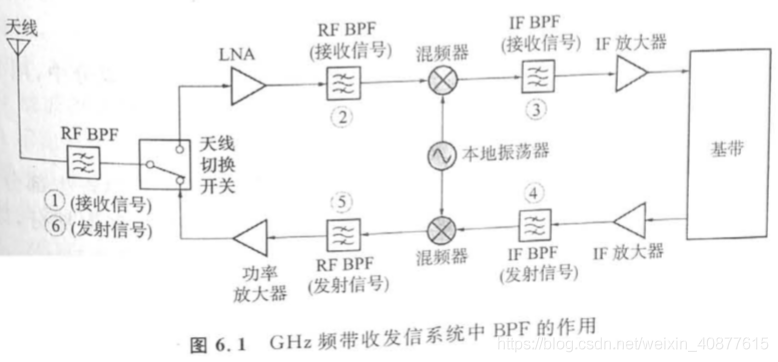①：RF BPF：从天线中取出期望频带的有效信号，滤除不需要频带的电磁波信号和噪声。 ②：RF BPF：用下级混频器(降频混频器)进行频率转换时，防止在IF频带中引入干扰信号，用BPF将不期望频率成分滤除。 ③：IF BPF：从混频器进行频率转换所产生的许多频率成分中，用BPF只取出期望的IF信号，也具有限制接收信号频带的作用。 ④：IF BPF：用混频器(升频转换器)进行频率转换时，用BPF防止在RF频带中引入干扰信号，也具有限制发射信号频带的作用。 ⑤：RF BPF：从混频器进行频率转换所产生的许多频率成分中，用BPF只取出期望的RF信号。 ⑥：RF BPF：用BPF除去功率放大器放大时失真所产生的寄生部分，以及大功率信号的输入在天线开关时所产生的寄生部分，以免寄生成分通过天线发射出去。
3 带通滤波器(BPF)选择 (1)对于低频电路，选择LC滤波器。LC滤波器通常用于基频领域。 (2)对于100MHz~1000MH频率范围，选择SAW滤波器。SAW滤波器通常用于IF BPF领域。 (3)对于2GHz频率电路，选择介质滤波器。介质滤波器通常用于RF BPF领域。
4 带通滤波器(BPF)详解 核心思想：BPF基本上是谐振电路。谐振电路分为串联谐振电路和并联谐振电路，对于串联谐振电路，若L和C无损耗，则在谐振频率时阻抗变为零；对于并联谐振电路，谐振频率时阻抗变为无穷大。对于串联谐振电路可知，L越大，衰减特性越陡；对于并联谐振电路可知，L越小C越大，衰减特性越陡。 谐振电路的谐振频率：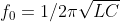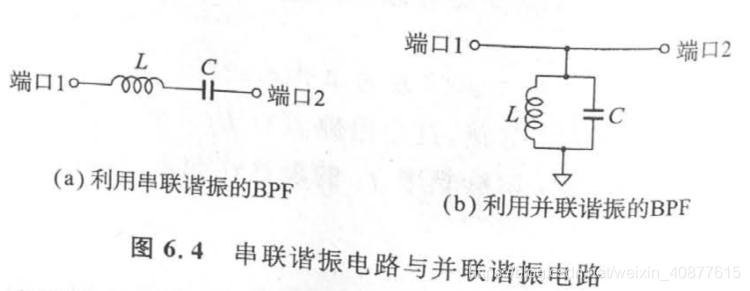串联谐振与并联谐振的组合电路：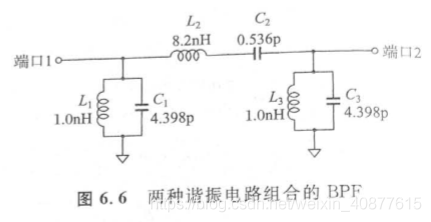5 介质滤波器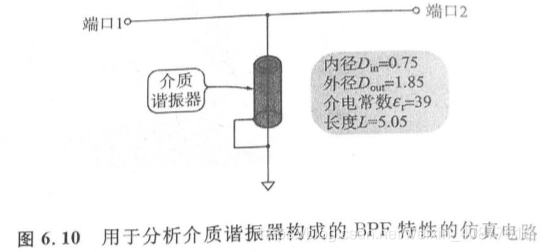6 微带线制作滤波器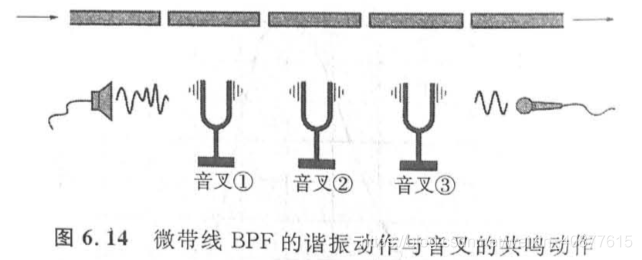三、部分源代码
clear all;
file_name = 'Data1.txt';    % 文件名
fid =fopen(file_name,'r');  %打开文件
h=fgets(fid);               %去掉第一行；
C=textscan(fid,'%*f%f%f%f%f');%去掉第一列；并将其他行列写入C
fclose(fid); %关闭文件
Data=[C{1}';C{2}';C{3}';C{4}'];%将C中的数据取出放入数组Data1
fid =fopen(['new_' file_name],'w'); %打开新文件夹
fprintf(fid,'%-.10e    %-.10e   %-.10e   %-.10e\r\n  ',Data); %将取出的数据放入新文件夹，纯数据
fclose(fid);%关闭新文件夹
t=['new_' file_name];

x1=newData(:,1);
x2=newData(:,2);
x3=newData(:,3);
x4=newData(:,4);

figure(1);
subplot(4,1,1)
plot(x1);
axis([0 512 0.5 1.7]);grid on;
% figure;
subplot(4,1,2)
plot(x2);
axis([0 512 0.5 1.7]);grid on;
% figure;
subplot(4,1,3)
plot(x3);
axis([0 512 0.5 1.7]);grid on;
% figure;
subplot(4,1,4)
plot(x4);
axis([0 512 0.5 1.7]);grid on;
N=512;
M=1; %调节因子

fs=1000;
%数据点数
%时间序列
n=0:N-1;
f=n*fs/N;    %频率序列
%%%%%%%%%%%%%%%%%%%%%%%%%%%%%%%%%%%%%%%%%%%%%%%
%信号
figure(2);

y1=fft(x1,N);    %对信号进行快速Fourier变换
mag1=abs(y1);     %求得Fourier变换后的振幅
subplot(4,1,1) ,plot(f(1:N/2),mag1(1:N/2));
axis([0 500 0 20]);grid on;

y2=fft(x2,N);    %对信号进行快速Fourier变换
mag2=abs(y2);    %求得Fourier变换后的振幅
subplot(4,1,2), plot(f(1:N/2),mag2(1:N/2));
axis([0 500 0 20]);grid on;

y3=fft(x3,N);    %对信号进行快速Fourier变换
mag3=abs(y3);     %求得Fourier变换后的振幅
subplot(4,1,3) ,plot(f(1:N/2),mag3(1:N/2));
axis([0 500 0 20]);grid on;

y4=fft(x4,N);    %对信号进行快速Fourier变换
mag4=abs(y4);     %求得Fourier变换后的振幅
subplot(4,1,4), plot(f(1:N/2),mag4(1:N/2));
axis([0 500 0 20]);grid on;
%=====%part3%===========%带通滤波器%========%
Wp = [50 150]/1000; Ws = [10 500]/1000;
Rp = 1; Rs =50;
[n,Wn] = buttord(Wp,Ws,Rp,Rs)
[b1,a1] = butter(n,Wn);
figure(3)
freqz(b1,a1)
title('n=4 Butterworth Bandpass Filter');
%=====%part3%===========%带通滤波器%========%
%=====%part4%===========%50hz陷波器%=======%
Wp = [10 500]/1000; Ws = [49 51]/1000;
Rp = 1; Rs = 50;
[n,Wn] = buttord(Wp,Ws,Rp,Rs)
[b2,a2] = butter(n,Wn,'stop');
figure(4)
freqz(b2,a2)
title('n=2 Butterworth Bandstop Filter');
%=====%part4%===========%50hz陷波器%=======%
%=====%part5%=============%数字滤波%========%
x1=filter(b1,a1,x1);
x1=filter(b2,a2,x1);
x2=filter(b1,a1,x2);
x2=filter(b2,a2,x2);
x3=filter(b1,a1,x3);
x3=filter(b2,a2,x3);
x4=filter(b1,a1,x4);
x4=filter(b2,a2,x4);

figure(5);
subplot(4,1,1)
plot(x1);grid on;
axis([0 512 -0.5 0.5])
subplot(4,1,2)
plot(x2);grid on;
axis([0 512 -0.5 0.5])
subplot(4,1,3)
plot(x3);grid on;
axis([0 512 -0.5 0.5])
subplot(4,1,4)
plot(x4);grid on;
axis([0 512 -0.5 0.5])

%=====%part5%=============%数字滤波%========%
figure(6);
y1=fft(x1,N);    %对信号进行快速Fourier变换
mag1=abs(y1);     %求得Fourier变换后的振幅
subplot(4,1,1) ,plot(f(1:N/2),mag1(1:N/2));
axis([0 500 0 11]);
grid on;

y2=fft(x2,N);    %对信号进行快速Fourier变换
mag2=abs(y2);    %求得Fourier变换后的振幅
subplot(4,1,2), plot(f(1:N/2),mag2(1:N/2));
axis([0 500 0 10]);
grid on;

y3=fft(x3,N);    %对信号进行快速Fourier变换
mag3=abs(y3);     %求得Fourier变换后的振幅
subplot(4,1,3) ,plot(f(1:N/2),mag3(1:N/2));
axis([0 500 0 10]);
grid on;

y4=fft(x4,N);    %对信号进行快速Fourier变换
mag4=abs(y4);     %求得Fourier变换后的振幅
subplot(4,1,4), plot(f(1:N/2),mag4(1:N/2));
axis([0 500 0 10]);
grid on;
%=====%part6%=============%验证效果%========%
iemg1=sum(abs(x1))/length(x1);
iemg2=sum(abs(x2))/length(x2);
iemg3=sum(abs(x3))/length(x3);
iemg4=sum(abs(x4))/length(x4);

四、运行结果五、matlab版本及参考文献
1 matlab版本 2014a
2 参考文献  沈再阳.精通MATLAB信号处理[M].清华大学出版社，2015. 高宝建,彭进业,王琳,潘建寿.信号与系统——使用MATLAB分析与实现[M].清华大学出版社，2020. 王文光,魏少明,任欣.信号处理与系统分析的MATLAB实现[M].电子工业出版社，2018.
展开全文人工智能
• 基于matlab的GUI应用的代码，包含音频文件的滤波程序（包含低频、高频以及带通），以及音频文件的合成。
• matlab带通滤波器，可以很好的实现带通滤波
• bandpass带通滤波详解基本使用适用范围注意事项原理解释 基本使用 先上代码，下面是带通滤波器在matlab中的使用 function [ pc ] = m_bandpass( pc ) freq = [0.1 0.5]; fs= 50; % Fa=Fs/2，Fa是分析频率 ...
• 使用matlab实现的同态滤波，高通巴特沃斯滤波，低通巴特沃斯滤波
• 自己编写的小程序，matlab程序，带通滤波器，可设置最小截止频率和最大截止频率。 ps。不附带信号文件，很简单的原理，旨在指导带通滤波器的编程思路与原理
• 它使用频域中的矩形窗口对时间序列进行带通滤波，“无需外部工具箱”。 下面给出了一个示例代码，用于过滤 200~300 Hz 通带中的时间序列： Fs = 1000； t = linspace(0,1,Fs); x = cos(2*pi*100*t)+0.5*randn(size(t...
• 设计带通滤波器，MATLAB程序.这个程序简单明了，能够很容易的进行滤波
• 最近有一个需求，在C#中实现FIR滤波，网上查了些资料感觉FIR滤波使用的还算比较多，相关的原理也比较简单。参考下面在Python环境中实现FIR的博客，在C#的环境中实现了一遍。 ...C#
• matlab实现的2D高斯带通滤波器，支持2D时间序列滤波
• 巴特沃斯带通滤波器的matlab代码图像处理器 空间领域 亮度 对比 3x3低通 5x5低通 7x7低通 9x9低通 边缘检测高通 高升 整体直方图均衡 自适应直方图均衡 频域 低通-理想 低通-高斯 低通-巴特沃思 高通-理想 高通-高斯...
• matlab开发-用于图像处理的高斯带通滤波器。此函数在频域中执行高斯带通滤波
• 框架已经写好 只需要更改 m q w0这些值 可求的S11 S12的曲线
• matlab匹配滤波代码时间分辨网络 如果在您的工作中使用此代码，请引用“时空灵活的子网揭示人脑中整合和分离的准循环性质”，Strindberg等人2021 如Strindberg等人2021中所述，TimeResolvedNets.m创建具有时间分辨的...
• 最近在处理数据过程中想着将数据进行一下滤波，所以就在查找资料同时记录一下学到的东西。在MATLAB中用带通滤波器滤波
• 带通滤波器——巴特沃斯滤波器为例matlab给出的代码如下：[n,Wn] = buttord(Wp,Ws,Rp,Rs)是不是显得很不人性化，即便是看了help中的英文解释，仍旧是对输入输出参数一知半解~那么我就用简单的语言来描述一下~首先...
• ## Matlab 实现信号滤波

万次阅读 多人点赞 2020-11-30 10:07:26
文章目录项目介绍代码实现1、导入信号2、加入噪声3、绘制原始信号的时域、频域4、滤波4.1 移动平均滤波4.2 中值滤波4.3 维纳滤波4.4 自适应滤波4.5 巴特沃斯滤波4.5.1 低通滤波4.5.2 高通滤波4.5.3 带通滤波 ...filter 信号处理
• 巴特沃斯带通滤波器的matlab代码心电图过滤器 心电图滤波器的Octave / Matlab实现，该滤波器专门用于过滤给定的心电图数据，其中包含60 Hz电力线噪声以及一些其他未指定的噪声。 编写此代码是为了完成万隆技术学院的...
• 对信号data实现20-350Hz的带通滤波，信号data的采样频率为Fs，代码如下： fp1=[50，300]；fs1=[20, 350]; Fs2=Fs/2; Wp=fp1/Fs2; Ws=fs1/Fs2; Rp=1; Rs=30; [n,Wn]=cheb2ord(Wp,Ws,Rp,Rs); [b1,a1]=cheby2(n,Rs,Wn);...信号处理
• Matlab实现带通滤波器，对于研究带通采样定理很有好处的。
• DATA_for_bandpass=data1; % 选择要分析的数据 f1=0.001; f2=1; f3=15; f4=20; for j=1:size(DATA_for_bandpass,2) data_bandpass(:,j)=bandpass_filter(DATA_for_bandpass(:,j),dt,f1,f2,f3,f4);...
• 图像处理的matlab代码Matlab实现图像高通滤波
• 在前一段时间遇到了一个MATLAB带通滤波方面的问题，滤波后首尾段数据都出现异常状况，然后就开始疯狂的查资料，但是这方面的资料说实话确实很少，尤其是这种滤波器性能导致的问题，后来偶然的巧合下，在MATLAB中文...异常处理
• matlab带通代码BCI_EEGNet 2 个 BCI 竞赛数据集的 EEGNet 实现： Kaggle 竞赛数据集： BCI 竞赛 III 数据集 2： EEGNet CNN 架构 PyTorch 实现借鉴自：Sriram Ravindran： 代码中使用的所有数据都较早在 MATLAB 中...
• 1. 图片读取，显示，存储 data = imread('test.jpg'); imshow(data); rgb=imread(inFile); % image(rgb) data=rgb; subplot(311); imshow(data); gdata = rgb2gray(data); % Filename:...n = graythres图像识别
• matlab声音过滤代码Matlab / octave文件用于RF设计中的实验方法 diode.m-二极管模型，简化和“最佳”二极管模型之间的差异。 绘制最佳二极管模型，二极管电阻和简化模型。 sound_to_FFT.m-接受声音文件并将内容转换......

# matlab带通滤波代码matlab 订阅